• 锐化处理的目的：是突出图像中的细节或者增强被模糊了的细节. 锐化处理可以用空间微分来完成. 微分算子的响应强度与图像在该点的突变程度有关，图像微分增强了边缘和其他突变(如噪声)而消弱了灰度变化缓慢的区域. ...
锐化处理的目的：是突出图像中的细节或者增强被模糊了的细节.
锐化处理可以用空间微分来完成. 微分算子的响应强度与图像在该点的突变程度有关，图像微分增强了边缘和其他突变(如噪声)而消弱了灰度变化缓慢的区域.
我们最感兴趣的是微分算子在①恒定灰度区域(平坦段)、②突变的开头与结尾(阶梯与斜坡突变)以及③沿着灰度级斜坡处的特性
对于一阶微分必须保证：
1.平坦段微分值为零
2.在灰度阶梯或斜坡的起点处微分值非零
3.沿着斜坡面微分值非零
对于二阶微分必须保证:
1.在平坦区微分值为零
2.在灰度阶梯或斜坡的起始点处微分值非零
3.沿着斜坡面微分值为零拉普拉斯算子定义为：拉普拉斯模板可以是：也可以是：拉普拉斯微分算子强调图像中灰度的突变,弱化灰度慢变化的区域。这将产生一幅把浅灰色边线、突变点叠加到暗背景中的图像。
将原始图像和拉普拉斯图像叠加在一起的简单方法可以保护拉普拉斯锐化处理的效果，同时又能复原背景信息。因此拉普拉斯算子用于图像增强的基本方法如下:可以用下面的掩模一次扫描来实现：代码如下：
img = imread('');
figure;
imshow(img);
title('原图')
i = 5;
w = fspecial('log', [i i], 0.5);%生成高斯-拉普拉斯滤波器
img_ruihua = filter(img, w, i);
figure;
imshow(img_ruihua);
tit = ['当前模板大小为' num2str(i)];
title(tit);

%%
%滤波函数
function img_result = filter(img, w, i)
moban_size = i;
[M,N] = size(img);
img_lap = zeros(M, N);
expand_img = double(wextend('2D','zpd', img, floor(i/2)));%扩展0，转double为了矩阵运算

for i=1:M
for j=1:N
ave = sum( sum( expand_img(i:i+moban_size-1,j:j+moban_size-1) .* w)); %取出扩展元素与模板相乘,并求矩阵元素之和
img_lap(i,j) = ave;
end
end

img_lap = uint8(img_lap);%转int8，图像
figure;
imshow(img_lap);
title('边缘')
img_result = img - img_lap;
end

结果示例：
原图：边缘提取如下：增强后的图形：总结：
锐化处理可以用空间微分来完成. 微分算子的响应强度与图像在该点的突变程度有关，图像微分增强了边缘和其他突变(如噪声)而消弱了灰度变化缓慢的区域。


展开全文opencv 边缘检测 matlab 计算机视觉
• 一、imfinfo函数——查看图像文件信息，注意参数是文件路径和文件名，不是图像对应的矩阵。 &gt;&gt; imfinfo('C:\Users\zhulf\Desktop\1.jpg') ans =   Filename: 'C:\Users\zhulf\Desktop\1.jpg'  ...
一、imfinfo函数——查看图像文件信息，注意参数是文件路径和文件名，不是图像对应的矩阵。

>> imfinfo('C:\Users\zhulf\Desktop\1.jpg')

ans =
Filename: 'C:\Users\zhulf\Desktop\1.jpg'
FileModDate: '19-Apr-2015 08:40:54'
FileSize: 1528413
Format: 'jpg'
FormatVersion: ''
Width: 1548
Height: 1548
BitDepth: 24
ColorType: 'truecolor'
FormatSignature: ''
NumberOfSamples: 3
CodingMethod: 'Huffman'
CodingProcess: 'Sequential'
Comment: {}
Make: 'Smartisan'
Model: 'Smartisan T1'
Orientation: 0
DateTime: '2015:04:19 08:40:53'
DigitalCamera: [1x1 struct]
GPSInfo: [1x1 struct]
MeteringMode: 'unknown'
LightSource: 'unknown'
Flash: 16

二、imshow函数

1、imshow(I)  显示图像I

Matlab代码：

>> imshow(I)

显示结果2、imshow(I,[low high])  它显示的是像素处理后的图像I，注意的是它只是显示的时候改变了图像像素，实际上并没有改变图像像素，图像像素值还是原来的值。

Matlab代码：

>> imshow(I,[0 80])它对图像像素的处理是：将I中像素值大于等于high变成high，将小于等于low的变成low，再将[low,high]中间的像素映射为默认的像素级，一般为[0,255]。

我们把上述描述转为代码：

mmax=max(I(:));

mmin=min(I(:));

for i=1:256

for j=1:256

if I2(i,j)<=0 I2(i,j)=0;

elseif I2(i,j)>=80

I2(i,j)=255;

end

end

end

I3=I2;

for i=1:256

for j=1:256

I3(i,j)=255/80*I3(i,j);

end

end

imshow(I3)

显示图像结果为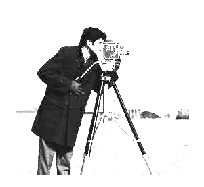和imshow(I,[0 80])效果是一样的。

3、 imshow(I,[ ])  它等同于imshow(I,[min(I:),max(I:)])，可以理解为把图像I转化成0-255灰度级来显示。

我们在这里做一个实验：下面三幅图分别为imshow(I)，imshow(I,[])和把图像像素值映射为[0,255]所显示的图像。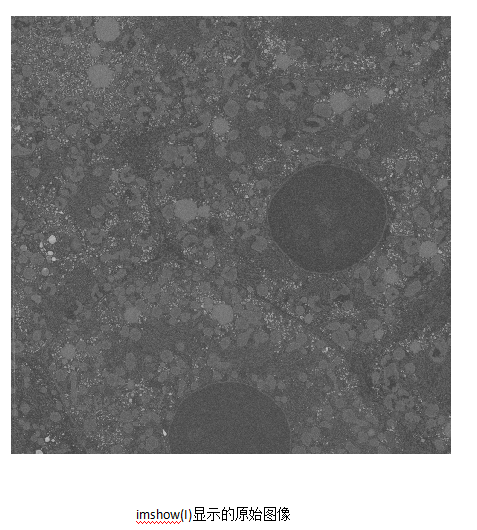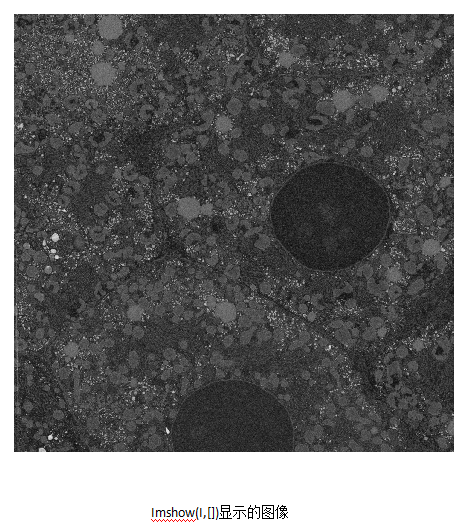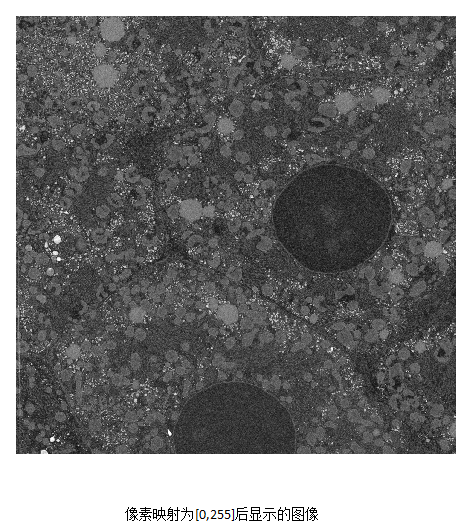我们发现，imshow(i,[])显示的效果正和把像素值映射为[0,255]效果所显示的一样。

Matlab代码如下：

mmax=max(A(:));

mmin=min(A(:));

for i=1:4096

for j=1:4096

A2(i,j)=1.0*255/(mmax-mmin)*(A(i,j)-mmin);

end

end

imshow(A)

figure,imshow(A,[])

figure,imshow(A2)

再例如，一个灰度级为17-2424的int16类型的图像，matlab显示(imshow(I))效果如下，可见，显示效果并不好，接下来我们使用imshow(I,[])，显示结果如下：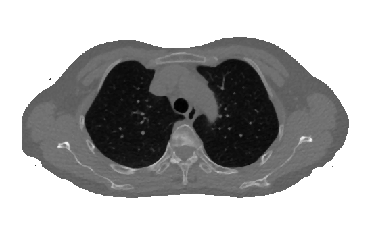显示结果较好，对比度较高。同样，imshow(I,[])的效果等同于把像素映射到0-255灰度级，映射后图像如下：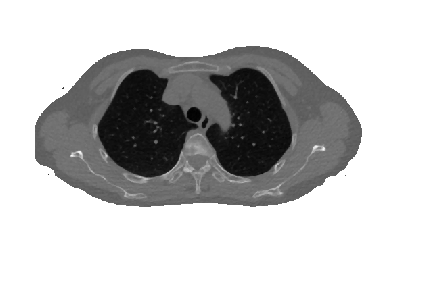实现灰度映射的Matlab如下：

mmax=double(max(A(:)));  %类型转换很重要

mmin=double(min(A(:)));  %类型转换很重要

for i=1:512

for j=1:512

tmp=double(A(i,j));  %类型转换很重要

A2(i,j)=uint8(255*(tmp-17)/2407);  %类型转换很重要

end

end

imshow(A)

figure,imshow(A,[])

figure,imshow(A2)

上面是对imshow()函数的理解，不明白的欢迎留言交流。

三、rgb2gray 将彩色图像转换为灰度图像

>> B=rgb2gray(A);
>> imshow(A)

>> figure,imshow(B)

四、数据类型转换函数

im2uint8  将图像转换为uint8类，相应的像素值也会变化到相应范围，如对uint16类图像应用im2uint8，则像素值由0-65535变化成0-255，这是一种线性映射。

im2uint16 将图像转换为uint16类，也有上面如此的性质。

im2double 将图像转化为double类，必要时缩放像素值。如果图像img是double型的，d=img;如果图像是logical或single型图像，d=double(img);如果图像是uint8型，d=double(img)/255；如果图像是uint16型，d=double(img)/65535。

im2double一般会将uint8归一化到[0,1],而double只是将数据变为double,如：

a = uint8(250);
b = double(a)
c = im2double(a)
b =
250
c =
0.9804

im2single 将图像转化为single类，性质和im2single类似。

mat2gray 可以将图像转换为标定[0,1]范围的double类的图像。

调用的语法是：

g=mat2gray(A,[Amin,Amax])，其中g具有0（黑）到1（白）的值。参数Amin使得A中小于Amin的值，在g中变为0；而在A中大于Amax的值，在g中变为1。

也可以是:

g=mat2gray(A)，

Amin,Amax默认为A中实际的最大值和最小值，它独立于输入的类，将整个输入值的范围标定为[0,1]。

>> f=[1 2;3 4]
f =
1     2
3     4
>> g=mat2gray(f)
g =
0    0.3333
0.6667    1.0000

BW = im2bw(I,LEVEL)   二值化图像，将大于LEVEL的像素值变为1，小于LEVEL的像素值变为0，且图像像素变成逻辑类；LEVEL是阈值，在[0,1]之间。

>> gb=im2bw(g,0.6)
gb =
0     0
1     1

>> gbv=islogical(gb)
gbv =
1

五、whos

B为图像矩阵

>> whos B
Name         Size                Bytes  Class    Attributes
B         1548x1548            2396304  uint8

六、反转图像

>> imshow(I)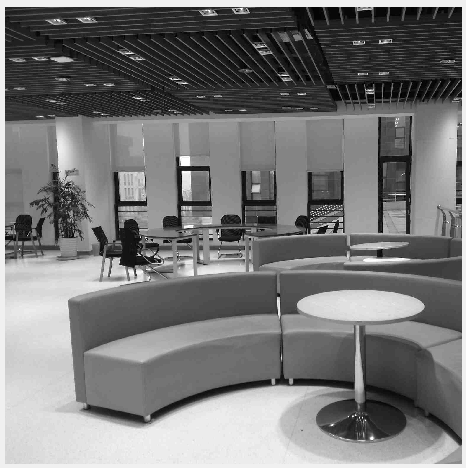上下翻转：

>>I2=I(end:-1:1,:);

>> figure,imshow(I2)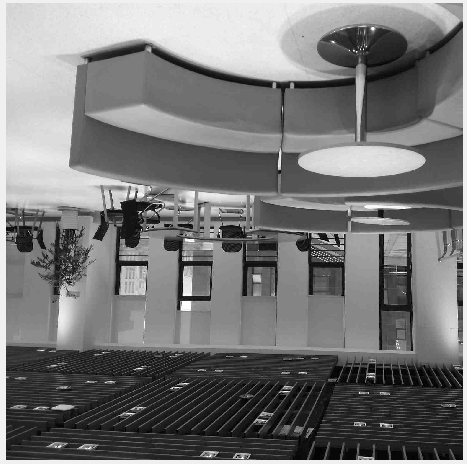上下左右均反转：

>> I3=I(end:-1:1,end:-1:1);
>> figure,imshow(I3)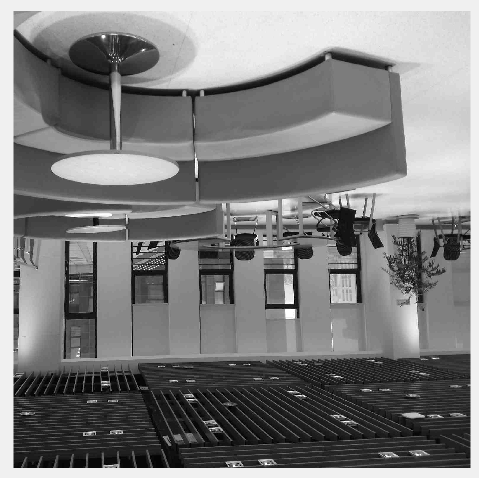七、抽取图像（压缩图像）

>> I4=I(520:855,90:837);
>> figure,imshow(I4)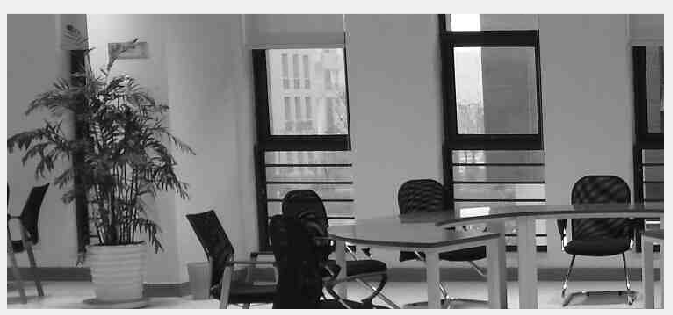图像也由原来的1548 x 1548变成了336 x 748。

>> I5=I(1:2:end,1:2:end);
>> figure,imshow(I5)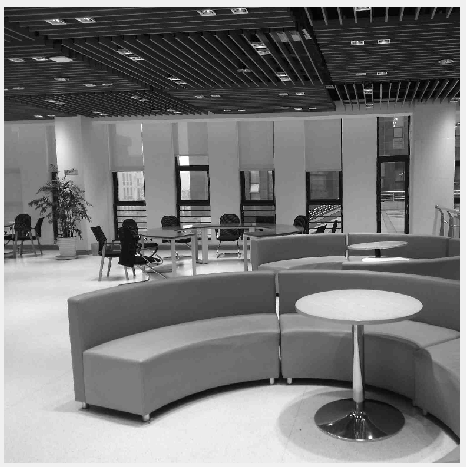图像由1548 x 1548 变成了774 x 774，filesize由99745变成了76747，做了部分压缩，但清晰度差不多。

八、improd()函数

function[p,pmax,pmin,pn]=improd(f,g)
%IMPROD Computes the produce of two images.
%[P,PMAX,PMIN,PN]=IMPROD(F,G) outputs the element-by-element
%product of two input images,F and G,the product
%maximum and minimum values,and a normalized product array with
%values in the rang[0,1].The input images must be of the same
%size.They can be of class uint8,uint16,or double. The outputs
%are of class double.
fd=double(f);
gd=double(g);
p=fd.*gd;
pmax=max(p(:));
pmin=min(p(:));
pn=mat2gray(p);

>> [p,pmax,pmin,pn]=improd(f,g)
p =
1     4
6     4
pmax =
6
pmin =
1
pn =
0    0.6000
1.0000    0.6000

之后陆续补充。。
展开全文matlab 图像处理
• 写在前头：说到数字图像处理，不得不提起MATLAB。这是一款非常方便的仿真软件，绝大多数的图像处理可以用MATLAB完成。 　有人问，处理图片，用PS岂不是更好。两者各有优点，如果需要将1００００幅图片转换成灰度...
写在前头：说到数字图像处理，不得不提起MATLAB。这是一款非常方便的仿真软件，绝大多数的图像处理可以用MATLAB完成。
有人问，处理图片，用PS岂不是更好。两者各有优点，如果需要将1００００幅图片转换成灰度图像并保存呢？MATLAB只需要一段很短的程序运行几秒就可以完成这个工作。
本文基于MatlabR2012a，将由浅入深写下去。

MATLAB中图像的基本操作

1、读取、显示图片

imread：
A = imread(filename, fmt)

A是结构体名，用来存储读入的图像数据。filename是读取的文件名，文件名要用”括起来。fmt是读取文件的类型如：jpg、png等等，这个参数可以不输入，由MATLAB自动判断。
显示函数imshow()原型：

    imshow：
imshow(I)

I为读取后保存在MATLAB中的结构体名。
程序实例：

A=imread('1.jpg');％读取名为１．ｊｐｇ的图片
imshow(A)％显示图片２、将灰度图片变成负片

对图像进行操作，实际上是将图像看成许多个像素点，对每个像素点进行操作。在计算机系统中，灰度图片被看成是许多个由值在［０～２５５］之间的像素点组成的图像，２５５表示白色，０表示黑色，黑白之间存在２５６个灰度级。负片是指将原灰度图白色的地方变成黑色，黑色的地方变成白色。也就是将０变成２５５，２５５变成０。ＭＡＬＴＡＢ的ｉｍａｄｊｕｓｔ（）函数提供了该功能。其函数原型：

imadjust：
J = imadjust(I,[low_in; high_in],[low_out; high_out])

其中，Ｉ为原灰度图像，ｌｏｗ＿ｉｎ，ｈｉｇｈ＿ｉｎ为输入图像的低和高灰度级，设置为［０，１］表示从０～２５５的归一化，ｌｏｗ＿ｏｕｔ，ｈｉｇｈ＿ｏｕｔ为输出图像的低高灰度级。
若是想将图片转换为负片，那么将[low_in; high_in]设置为［０，１］，将[low_out; high_out]设置为［１，０］。即原来输入为０的地方变成１输出，输入为１的地方变成０输出。
实例：

　A=imread('1.jpg');
imshow(A)％显示原图
figure,imshow(A1)％显示负片３、彩色图片转换成灰度图片

我们在计算机中看到的大多数彩色图片是基于ＲＢＧ三通道的图片，红绿蓝三种颜色，每一种取值均为［０～２５５］。通过２５５＊２５５＊２５５，可以构成庞大的颜色群。而灰度图像只有２５６个灰度级。通过以下公式可以将ＲＧＢ转换成灰度
ＧＲＡＹ＝0.2989  R + 0.5870 * G + 0.1140 * B*
ＭＡＴＬＡＢ中提供的函数ｒｇｂ２ｇｒａｙ为我们提供了将彩色图片转换成灰度图片的功能。函数原型：

I = rgb2gray(RGB)

实例：

 A=imread('001.png');％原彩色图片
imshow(A)
A_gray=rgb2gray(A);％转换成灰度图片
figure,imshow(A_gray)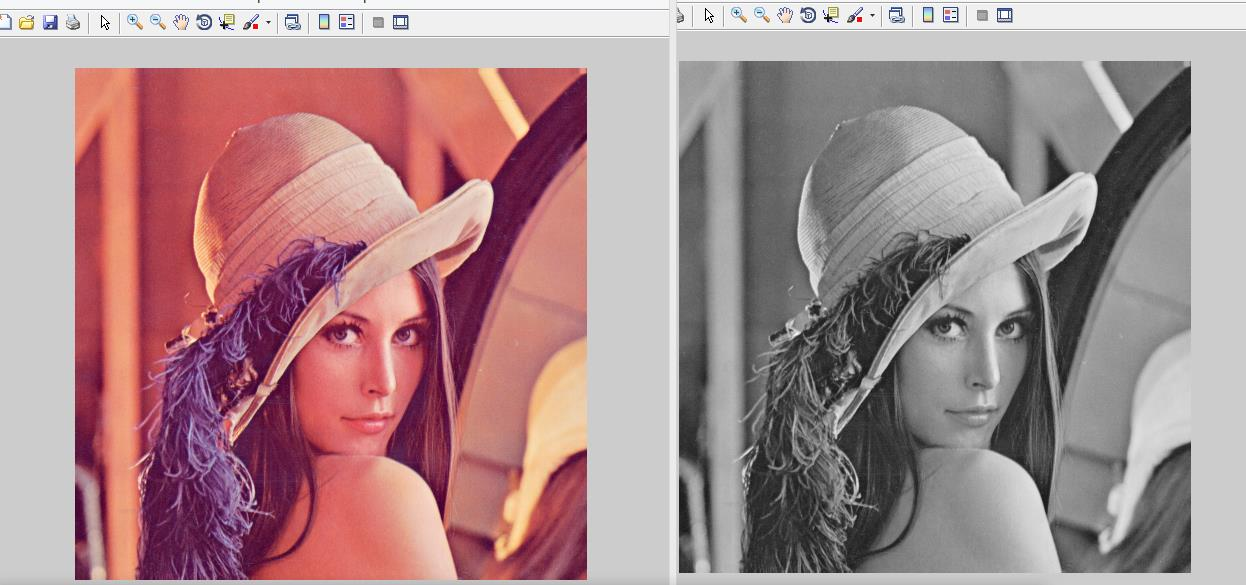展开全文图像处理 matlab 图片
• 平滑滤波——matlab图像处理 平滑滤波的目的是消除或尽量减少噪声，改善图像的质量。假设加性噪声是随机独立分布，这样利用图像像素领域的平均或加权平均即可有效地抑制噪声干扰。从信号分析的观点来看，图像平滑...
平滑滤波——matlab图像处理
平滑滤波的目的是消除或尽量减少噪声，改善图像的质量。假设加性噪声是随机独立分布，这样利用图像像素领域的平均或加权平均即可有效地抑制噪声干扰。从信号分析的观点来看，图像平滑本质上是低能滤波，信号的低频部分可通过，高频的噪声信号被阻截。但由于图像边缘也处于高频部分，这样往往带来另一个问题：在对图像进行平滑处理时，往往对图像的细化造成一定程度的损坏。
领域运算可用领域与模版的卷积得到，这极大地方便了计算。
MATLAB中提供的imfliter函数用于实现图像的平滑处理，其调用格式如下。
B=imfliter（A，H）：使用多维滤波器H对图像A进行滤波（平滑）。参数A可以是任意维的二值或非奇异数值型矩阵。参数H为矩阵，表示滤波器。H常由函数fspecial输出得到。返回值B与A的维数相同。
B=imfliter（A,H，optional1，optional2，…）

参数类型
说明

X
输入图像的外边界通过X来扩展，X默认值为0

symmetric
输入图像的外部边界通过镜像反射其内部边界来扩展

circular
输入图像的别界通过假设输入图像为周期函数来扩展

relicate
输入图像的外部别界通过复制内部别界的值来扩展

same
输入和输出图像大小相等，默认操作

full
输出图像比输入图像大

corr
使用相关进行滤波（平滑）

conv
使用卷积进行滤波（平滑）

matlab中提供的fspecial函数用于创建二维滤波器：
h=fspecial（type）：
type可以是：average，disk，gaussian，laplacian，log，motion，prewitt，sobel，unsharp。
h=fspecial（type，parameters）：创建指定类型和指定参数的二维滤波器h。参数parameters为与滤波器有关的参数。
clear all;
subplot(2,2,1);imshow(I);
xlabel('(a)原始图像');
H = fspecial('motion',20,45);
MotionBlur = imfilter(I,H,'replicate');
subplot(2,2,2);imshow(MotionBlur);
xlabel('(b)运动模糊图像');
H=fspecial('disk',10);
blurred = imfilter(I,H,'replicate');
subplot(2,2,3);imshow(blurred);
xlabel('(c)模糊图像');
H=fspecial('unsharp');
sharpened = imfilter(I,H,'replicate');
subplot(2,2,4);imshow(sharpened);
xlabel('(d)锐化图像');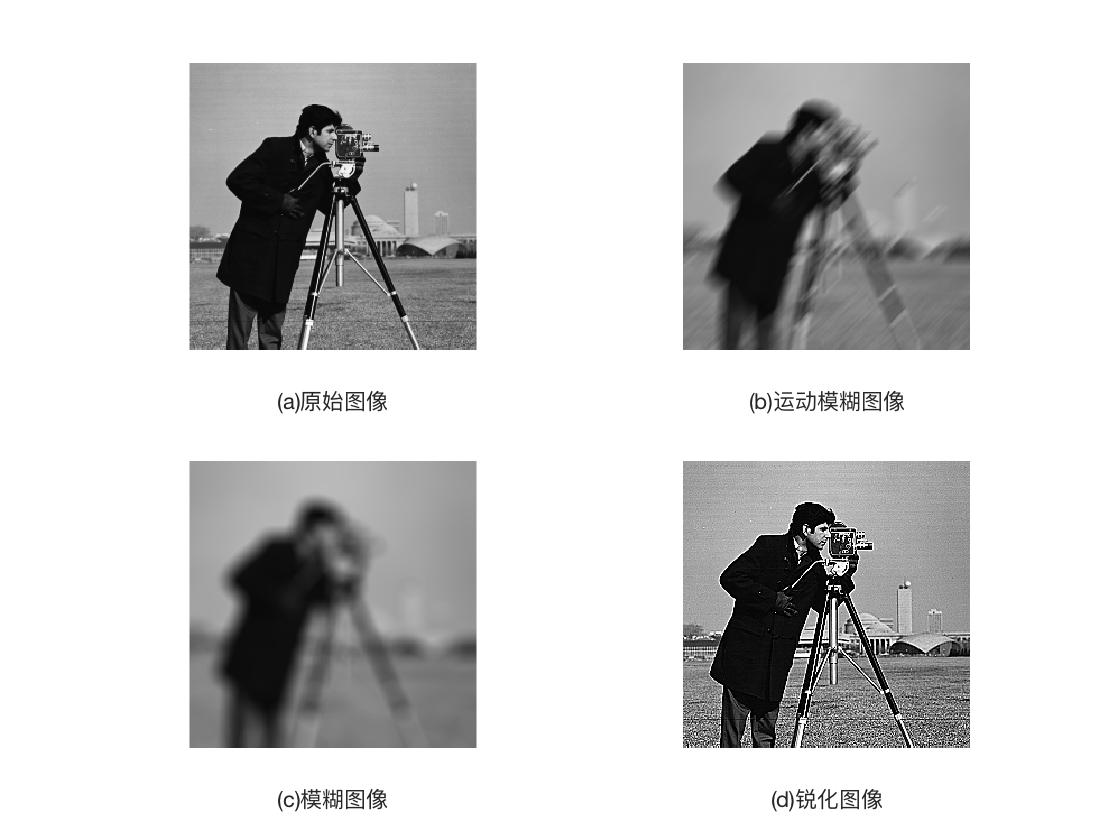对含有高斯噪声的图像进行平滑处理。
clear all;
Inoised = imnoise(I,'gaussian',0.1,0.005);%对图像进行高斯噪声加噪
%制定卷积核
h=ones(3,3)/5;
h(1,1) = 0;
h(1,3) = 0;
h(3,1) = 0;
h(1,3) = 0;
%平滑运算
I2=imfilter(Inoised,h);
subplot(1,3,1);imshow(I);
xlabel('(a)原始图像');
subplot(1,3,2);imshow(Inoised);
xlabel('(b)带噪声图像');
subplot(1,3,3);imshow(I2);
xlabel('(c)平滑后图像');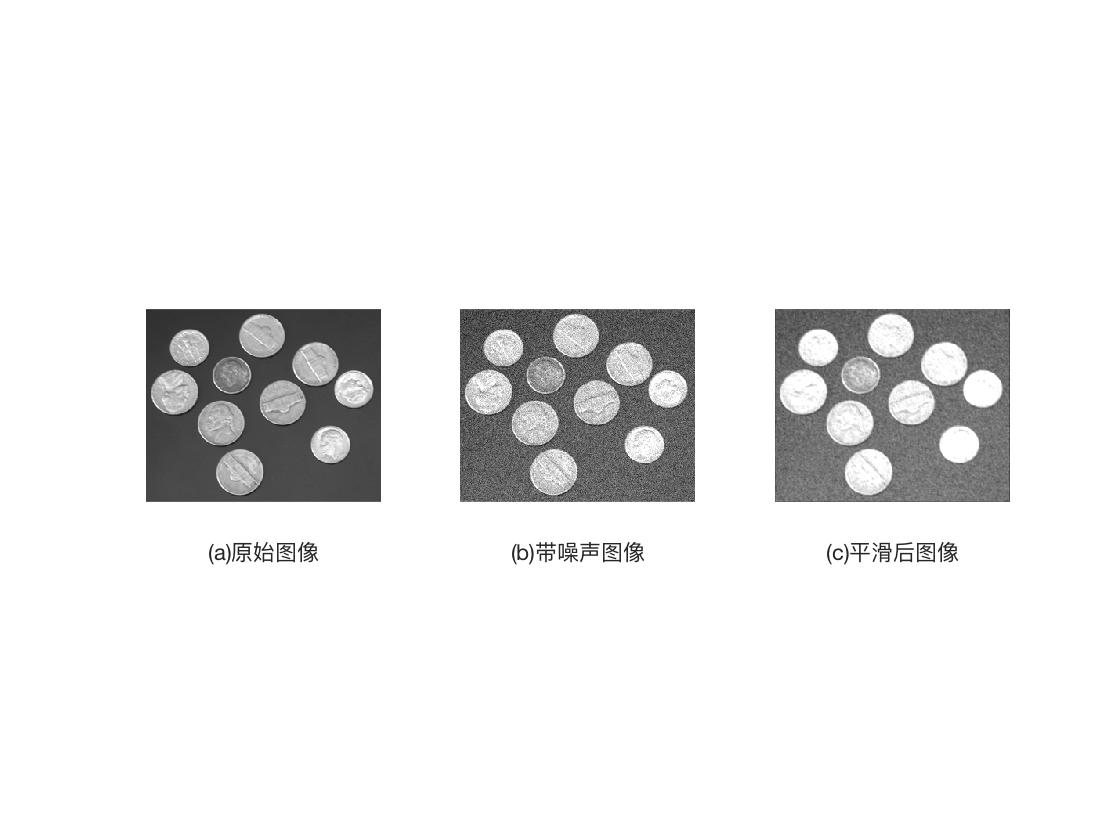有问题还请多多指教。刚刚入门。谢谢各位大牛。


展开全文平滑滤波 Matlab
• MATLAB图像处理课程
MATLAB图像处理—2950人已学习 课程介绍MATLAB图像处理课程课程收益    通过学习该课程系统掌握MATLAB图像处理的相关知识点。讲师介绍    李立宗更多讲师课程    南开大学计算机硕士毕业，目前在天津本地一所高校任副教授。课程大纲  第1章:几何变换    1.几何变换  14:23  第2章:正交变换    1.离散余弦变换  5:42    2.傅里叶变换  6:28    3.离散小波变换  10:05    4.提升小波变换  8:06  第3章:边缘检测    1.边缘检测  5:35  第4章:形态学变换    1.图像腐蚀  5:33    2.图像膨胀  4:40    3.开运算  4:49    4.闭运算  4:47  第5章:图像增强    1.直方图  7:05    2.灰度变换  7:40    3.灰度对数变换  4:52    4.中值滤波  3:42  第6章:JPEG压缩    1.JPEG压缩  5:36  第7章:类型转换    1.RGB转灰度  3:02    2.灰度图像二值化  3:21  第8章:图像滤波    1.图像滤波  7:10  第9章:图像裁剪    1.图像裁剪  5:57  第10章:噪声    1.简述  5:31    2.高斯噪声  3:16    3.localvar噪声-语法格式1  6:29    4.localvar噪声-语法格式2  8:10    5.泊松噪声  3:06    6.椒盐噪声  5:48    7.speckle噪声  5:12  第11章:图像抖动    1.图像抖动  2:29  第12章:数字水印    1.基本原理  7:20    2.具体实现  8:33  第13章:图像融合    1.加法融合  4:08    2.小波融合  5:53    3.小波大数融合（理论讲解）  5:21    4.小波大数融合（代码讲解）  6:04  第14章:混沌系统    1.系统概述  4:14    2.绘制序列  7:00    3.初始值敏感性  5:58    4.二值化处理  7:27    5.生成二值混沌图像  8:40    6.生成灰度混沌图像  8:59    7.生成彩色混沌图像  9:38大家可以点击【查看详情】查看我的课程
展开全文图像处理 matlab
• 显示索引图像和灰度图像&gt;&gt; [X,map]=imread('trees.tif');&gt;&gt; gmap=rgb2gray(map);&gt;&gt; figure,imshow(X,map);&gt;&gt; figure,imshow(X,gmap);利用膨胀函数平移图像...matlab函数 数字图像处理函数
• Matlab图像处理新手——图像分割和画框显示篇 **前言：**许多人说Matlab对未来的工作没什么用，就目前了解的情况而言并非如此。如：现版本已有matlab转化成C语音的功能；matlab与DSP（API协议技术）、FPGA、西门子...
• 计算机图像处理实验课1-9，看完本课程可以让你matlab技能小小进步，对图像处理稍微了解。、 （一）matlab数字图像处理实验-guide的简单使用教程 （二）matlab数字图像处理实验-图像的几何变换 （三）matlab数字...matlab 图像处理
• 自己在学习过程中查阅资料整理了MATLAB图像处理工具箱中函数的名称列表，以及MathWorks中讲解各个函数使用的链接： 1.绘图工具箱 plot 基本绘图函数 mesh 绘制三维网格曲面 fplot 绘制数学函数图 ....matlab 图像处理
• 在MATLAB中，基本数据结构为数列，大部分图像也是以数列的方式存储的，例如，包含1024列768行的彩色图像，在MATLAB中被...1.MATLAB图像表达方式 在MATLAB中，图像可以以两种方式表达，分别为像素索引和空间位置。 （1）
• zzfrom: http://public.whut.edu.cn/lxy/mathmodel/Resource/course/Matlab%206.0图形图像处理函数详细参考.pdf1.applylut 功能: 在二进制图像中利用lookup表进行边沿操作. 语法: A = applylut(BW,lut) 举例 lut = ...matlab 图像处理 filter
•图像处理 matlab
• Matlab图像处理大师淘宝店（QQ:3249726188） 专注、专一于Matlab图像处理 本店绝非中介，本店代码均由本人及硕士师弟代写完成，提供有偿Matlab算法代码编程服务，不从事不违反涉及学术原则的事。 另外，本店的...算法分析
• 1.2图像的表示方法 常见的5种图像表示方法:分别是二进制图像,索引图像,灰度图像,RGB图像和多帧图像 1.2.1二进制图像(二值图像) 通常由一个二维数组表示,一位表示一个像素.非0即1,0表示黑色,1表示白色 1.2.2 灰度...matlab 数字图像处理
• 计算机视觉/图像处理研究中经常要用到Matlab，虽然其自带了图像处理和计算机视觉的许多功能，但是术业有专攻，在进行深入的视觉算法研究的时候Matlab的自带功能难免会不够用。本文收集了一些比较优秀的Matlab计算机...matlab 图像处理
• ①目前，数字图像处理的信息大多是二维信息，处理信息量很大。 ②数字图像处理占用的频带较宽。 ③数字图像中各个像素是不独立的，其相关性大。3.什么是像素？ 像素是一个面积概念，是构成数字图像的最小单位。4....matlab
• GUI，即图形用户接口，是MATLAB的可视化操作功能，本文编写一个简单GUI进行图像处理。1、新建GUI文件，点击file-&gt;new-&gt;GUI如下图所示点击GUI后出现如下图界面，默认为Blank GUI (Default)选项，点击OK...MATLAB 图像处理 GUI
• 今天发现了matlab图像处理工具箱的强大之处， 任务缘由： 需要对图像进行批处理改变尺寸 一般遇到这种任务，我们往往都会写matlab代码进行批量操作 但是matlab有自带的很强大的图像处理工具箱，  图片批处理...matlab图像处理
• 1、win10）环境MATLAB2017a 百度云链接失败，可能被屏蔽了。 安装及破解方法： 下载完--选中--所有压缩包一起解压，出现如下文件--打开--里面有两个.iso文件，分别解压 然后新建一个文件：R2017a_win64_...MATLAB GUI 图像处理
...